We think you are located in Nigeria. Is this correct?

# Test yourself now

High marks in maths are the key to your success and future plans. Test yourself and learn more on Siyavula Practice.

# Chapter 6: Algebraic expressions

Last year you learnt that an algebraic expression is a mathematical phrase or statement that contains numbers, symbols, variables (such as $x$ or $q$) and operators (such as $+$, $-$, $\times$ and $\div$). Note that algebraic expressions do not have equals signs.

Algebraic expressions are made up of terms that are separated by an addition ( $+$) or a subtraction $(-)$ sign. The algebraic expression $2x+1$ has two terms. The algebraic expression $2a + b - 6c + 4$ has four terms.

A number multiplied by a variable in a single term of an algebraic expression is called a coefficient. A constant is a term in an algebraic expression that does not contain a variable. Coefficients and constants are directed numbers; they can be positive or negative.

algebraic expression An algebraic expression is a mathematical statement that contains a combination of numbers, symbols, variables and mathematical operators. It does not have an equals sign.

term A term can be a single number (positive or negative), or a variable, or one or more numbers and variables that are being multiplied together. Terms are separated by $+$ or $-$ signs.

coefficient A coefficient is a number multiplied by a variable in a single term of an algebraic expression.

constant A constant is a term in an algebraic expression that does not contain a variable.

The following example summarises the terminology you learnt last year:

• There are three terms: $3x$, $-5y$ and $9$.
• The variables are $x$ and $y$.
• The coefficient of $x$ is $3$.
• The coefficient of $y$ is $-5$.
• The constant term is $9$.

## 6.1 Simplifying algebraic expressions

In Chapter 5 you learnt how to multiply and divide directed numbers. Remember:

• $(+)\times(+)=(+)$
• $(-)\times(-)=(+)$
• $(+)\times(-)=(-)$
• $(-)\times(+)=(-)$

• $\frac{(+)}{(+)}=(+)$
• $\frac{(-)}{(-)}=(+)$
• $\frac{(+)}{(-)}=(-)$
• $\frac{(-)}{(+)}=(-)$

When you multiply or divide positive and negative numbers, the general principle is:

• The same signs give a positive answer.
• Opposite signs give a negative answer.

### Multiplying and dividing variables

From previous grades you know that we determine the area of a square using the formula:

Consider the following square:The area of the square is calculated as follows:

\begin{align} \text{area} & = \text{side} \times \text{side} \\ & = 60\text{ mm} \times 60\text{ mm} \\ & = 60^2\text{ mm}^2 \\ & = 3,600 \text{ mm}^2 \end{align}

Similarly, the area of the square below is: $b \times b = b^2$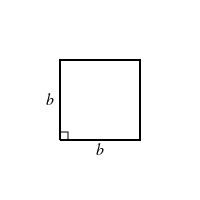### Worked example 6.1: Multiplying variables

Simplify:

1. Step 1: Multiply the coefficients. Remember that the plus or minus sign is part of the coefficient.

2. Step 2: Multiply the variables.

From the squares above the worked example, you know that $x \times x = x^2$. Therefore:

\begin{align} x \times xy &= x \times x \times y \\ &= x^2 \times y \\ &= x^2y \end{align}
3. Step 3: Combine the answers from Steps 1 and 2.

Last year you learnt that we can simply a fraction by dividing the numerator and denominator by the same number. For example:

Now that you understand how this works, you may write this in a shorter way. The Highest Common Factor (HCF) of 12 and 16 is 4. Therefore:

If necessary, go back to Chapter 1 to revise how to determine the HCF of two or more numbers.

We can apply the same method when dividing variables. For example,

is simplified as follows:

\begin{align} \frac{x^2y}{x} & = \frac{x \times x \times y}{x} \\ & = \frac{\cancel{x} \times x \times y}{\cancel{x}} \\ & = \frac{1 \times x \times y}{1} \\ & = x \times y \end{align}
• It is incorrect to say that we "cancel out" the variable $x$. We are dividing the numerator and the denominator by their HCF, which is $x$. Just as $\frac{5}{5} = \frac{1}{1} = 1$, the fraction $\frac{x}{x} = \frac{1}{1} = 1$.
• Remember that we do not write down the number 1 if it is a coefficient or a denominator. Therefore, $1x$ is written as $x$ and $\frac{x}{1}$ is written as $x$.

### Worked example 6.2: Dividing variables

Simplify:

1. Step 1: Find the HCF of the coefficients and simplify them.

The HCF of 4 and 10 is 2.

2. Step 2: Write out the variables and simplify them.

3. Step 3: Combine the answers from Steps 1 and 2. Decide what the sign of the answer must be.

A positive number divided by a negative number gives a negative number. Always write a minus sign in front of a fraction in its simplest form. Therefore:

### Exercise 6.1: Multiply and divide variables

Simplify the following algebraic expressions.

### Expanding algebraic expressions with brackets

When we expand an algebraic expression, we use the distributive law to remove brackets and then we collect like terms. You learnt the distributive law last year. If we have a term in an algebraic expression that has a bracket, we can multiply the number or variable before the bracket with all the terms inside the bracket. We can represent the distributive law as follows: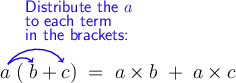expand We expand an algebraic expression by using the distributive law to remove brackets and then collecting like terms.

distributive law The distributive law states that the multiplying factor or coefficient of a bracket can be distributed across each of the terms inside the bracket, for example $a(b+c) = ab+bc$.

Here are two examples you did last year:

\begin{align} 4 \times (3 + 2) & = 4 \times 3+ 4 \times 2\\ & = 12 + 8 \\ & = 20 \end{align} \begin{align} 2 \times (a + b) & = 2 \times a + 2 \times b\\ & = 2a + 2b \end{align}

Remember that $a \times b$, $(a)(b)$, $a(b)$, $(a)b$ and $ab$ all mean $a$ multiplied by $b$.

We can represent the distributive law in terms of area. From previous grades you know that we determine the area of a rectangle using the formula:

Look at the rectangles below.

• For the red rectangle: $\text{area} = a \times b = ab$
• For the blue rectangle: $\text{area} = a \times c = ac$
• For the green rectangle: $\text{area} = a \times (b+c) = a(b+c)$

From the diagram, you can see that the area of the green rectangle is equal to the sum of the areas of the red and blue rectangles. Therefore: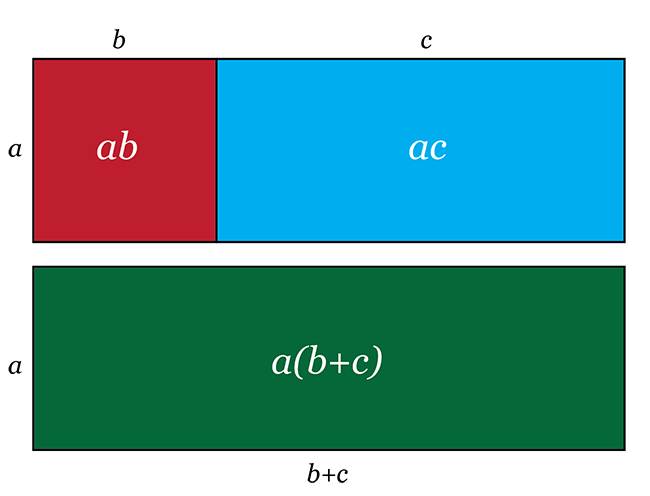### Worked example 6.3: Expanding algebraic expressions with one bracketed term

Expand and simplify:

1. Step 1: Identify the terms.

Terms are separated by plus or minus signs that are not inside a bracket. This expression has two terms.

2. Step 2: Apply the distributive law to the term with the brackets.

Remember that the minus sign in front of the 5 is part of the coefficient of the bracket. So you need to multiply each time by -5.

\begin{align} 6-5(x+y-2x)&=6\,\color{blue}{-\,5 \times x} \color{green}{\,-\,5\times \! +y} \color{orange}{\,-\,5\times \! -2x} \end{align}
3. Step 3: Simplify by following the correct order of operations.

Remember the correct order of operations is: Brackets, Of, Division, Multiplication, Addition, Subtraction.

\begin{align} 6-5(x+y-2x)&=6\,\color{blue}{-\,5 \times x} \color{green}{\,-\,5\times \! +y} \color{orange}{\,-\,5\times \! -2x}\\ &= 6-5x-5y+10x \\ \end{align}
4. Step 4: Collect like terms.

\begin{align} 6-5(x+y-2x)&=6\,\color{blue}{-\,5 \times x} \color{green}{\,-\,5\times \! +y} \color{orange}{\,-\,5\times \! -2x}\\ &= 6-5x-5y+10x \\ &= -5x+10x-5y+6 \\ &= 5x-5y+6 \end{align}

By convention, we place the terms with variables first, and then the constant term.

### Exercise 6.2: Expand algebraic expressions with one bracketed term

Expand and simplify the following algebraic expressions.

1. \begin{align} \phantom{=} & \, 7m(2n-3m) \\ = & \, 7m\times 2n + 7m\times -3m \\ = & \, 14mn-21m^2 \end{align}
2. \begin{align} \phantom{=} & \, 6x+2(4-2x) \\ = & \, 6x+2\times4+2\times-2x \\ = & \, 6x+8-4x \\ = & \, 2x+8 \end{align}
3. \begin{align} \phantom{=} & \, -p(2p-q+1) \\ = & \, -p\times 2p -p\times -q -p \times +1 \\ = & \, -2p^2+pq-p \end{align}
4. \begin{align} \phantom{=} & \,(x-y+z)4+4y \\ = & \,x \times 4 -y \times 4 +z \times4 +4y \\ = & \,4x-4y+4z+4y \\ = & \,4x+4z \end{align}
5. \begin{align} \phantom{=} & \, 2(x+y)-3(x-y) \\ = & \, 2 \times x +2 \times y -3 \times x -3 \times -y \\ = & \, 2x+2y-3x+3y \\ = & \, -x+5y \end{align}

We can also apply the distributive law to algebraic expressions that have two bracketed terms. Look at the following diagram.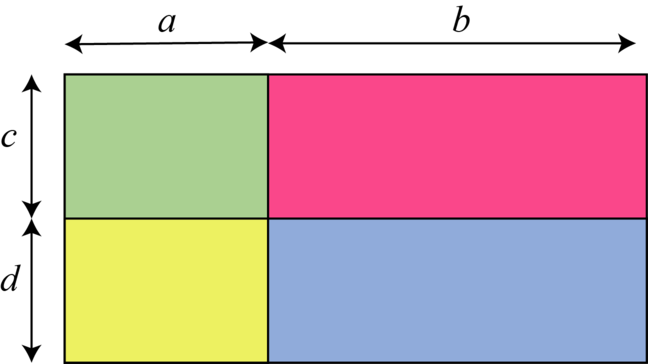• For the green rectangle: $\text{area}=a \times c = ac$
• For the yellow rectangle: $\text{area}=a \times d = ad$
• For the pink rectangle: $\text{area}=b \times c = bc$
• For the blue rectangle: $\text{area}=b \times d = bd$

If we add these four rectangles together, we get the area of the large rectangle, which is:

From this, it follows that:

We can represent this version of the distributive law as follows:If you use the method described in the following worked example when expanding two brackets, it will be to your advantage in later years.

### Worked example 6.4: Expanding algebraic expressions with two bracketed terms

Expand and simplify:

1. Step 1: Multiply the two terms that stand first in each bracket.

2. Step 2: Multiply the two terms that stand on the outsides, that is, the furthest away from each other.

3. Step 3: Multiply the two terms that stand on the insides, that is, the closest together.

4. Step 4: Multiply the two terms that stand last in each bracket.

5. Step 5: Combine the answers from Steps 1 to 4.

6. Step 6: Collect like terms.

First term in each bracket
Outside terms
Inside terms
Last term in each bracket

### Exercise 6.3: Expand algebraic expressions with two bracketed terms

Expand and simplify the following algebraic expressions.

1. \begin{align} \phantom{=} & \, (7a+1)(3a+2) \\ = & \, 21a^2+14a+3a+2 \\ = & \, 21a^2+17a+2 \end{align}
2. \begin{align} \phantom{=} & \, (3x+y)(x-y) \\ = & \, 3x^2-3xy+xy-y^2 \\ = & \, 3x^2-2xy-y^2 \\ \end{align}
3. \begin{align} \phantom{=} & \, (5a-2b)(3a+b) \\ = & \, 15a^2+5ab-6ab-2b^2 \\ = & \, 15a^2-ab-2b^2 \end{align}
4. \begin{align} \phantom{=} & \, (4b-2c)(2b-4c) \\ = & \, 8b^2-16bc-4bc+8c^2 \\ = & \, 8b^2-20bc+8c^2 \\ \end{align}
5. \begin{align} \phantom{=} & \, 2+(2m-3)(6m-4) \\ = & \, 2+12m^2-8m-18m+12 \\ = & \, 12m^2-26m+14 \\ \end{align}

Look at the square below.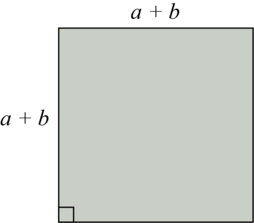The area of the square is given by:

\begin{align} \text{area}&=\text{side}\times\text{side} \\ &=(a+b)\times(a+b) \\ &=(a+b)^2 \end{align}

A bracket to the power two means that we have two identical brackets. We can then apply the distributive law to the brackets.

### Worked example 6.5: Expanding algebraic expressions with two identical bracketed terms (Method 1)

Expand and simplify:

1. Step 1: Write out the bracket that is given to the power two as two identical brackets.

2. Step 2: Apply the distributive law to the two brackets before distributing the coefficient.

Remember the acronym FOIL:
First term in each bracket
Outside terms
Inside terms
Last term in each bracket

Multiply the two terms that stand first in each bracket.

3. Step 3: Multiply the two terms that stand on the outsides, that is, the furthest away from each other.

4. Step 4: Multiply the two terms that stand on the insides, that is, the closest together.

5. Step 5: Multiply the two terms that stand last in each bracket.

6. Step 6: Combine the answers from Steps 2 to 5.

7. Step 7: Collect like terms.

8. Step 8: Apply the distributive law to the remaining bracket.

\begin{align} \phantom{=} & \, 4(2x-3y)(2x-3y) \\ = & \, 4(4x^2-12xy+9y^2)\\ = & \, 16x^2-48xy+36y^2 \end{align}

If you understand the method above, you may also use the shorter method shown in the worked example below. Note that the following method can only be applied to two identical brackets.

### Worked example 6.6: Expanding algebraic expressions with two identical bracketed terms (Method 2)

Expand and simplify:

1. Step 1: Apply the distributive law to the bracket given to the power two before distributing the coefficient. Square the term that stands first in the bracket.

2. Step 2: Multiply the two terms in the bracket by each other and by the number 2.

3. Step 3: Square the term that stands last in the bracket.

4. Step 4: Combine the answers from Steps 1 to 3.

5. Step 5: Apply the distributive law to the remaining bracket.

Remember that the minus sign in front of the 4 is part of the coefficient that must be multiplied by each term in the bracket.

\begin{align} &-4(3a-5b)^2 \\ =&-4(9a^2-30ab+25b^2)\\ =&-36a^2+120ab-100b^2 \end{align}

### Exercise 6.4: Expand algebraic expressions with two identical bracketed terms

Expand and simplify the following algebraic expressions.

1. \begin{align} \phantom{=} & \, (x+5)^2 \\ =& \, x^2+x \times 5 \times 2 + 5^2 \\ =& \, x^2+10x+25 \end{align}
2. \begin{align} &-(m+n)^2 \\ =&-(m^2+m\times n \times 2 +n^2) \\ =&-(m^2+2mn+n^2) \\ =&-m^2-2mn-n^2 \end{align}
3. \begin{align} \phantom{=} & \, 5(2b-2c)^2 \\ =& \, 5((2b)^2+2b\times -2c \times 2 +(2c)^2) \\ =& \, 5(4b^2-8bc+4c^2) \\ =& \, 20b^2-40bc+20c^2 \end{align}
4. \begin{align} \phantom{=} &\, (p+q)^2-2(p+q)^2 \\ =&\, p^2+p \times q \times 2 +q^2 -2(p^2+p \times q \times 2+q^2) \\ =&\, p^2+2pq+q^2-2(p^2+2pq+q^2) \\ =&\, p^2+2pq+q^2-2p^2-4pq-2q^2 \\ =&\, -p^2-2pq-q^2 \end{align}
5. \begin{align} \phantom{=} & \, 8y^2-2(3x+4y)^2 \\ =& \, 8y^2-2((3x)^2+3x \times 4y \times 2 +(4y)^2) \\ =& \, 8y^2-2(9x^2+24xy+16y^2) \\ =& \, 8y^2-18x^2-48xy-32y^2 \\ =& \, -24y^2-48xy-18x^2 \end{align}

Suppose we want to determine the orange area in the diagram below.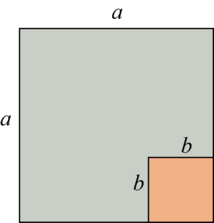The orange area is the area of the large square minus the area of the small square:

We can also determine the orange area by using the diagram below.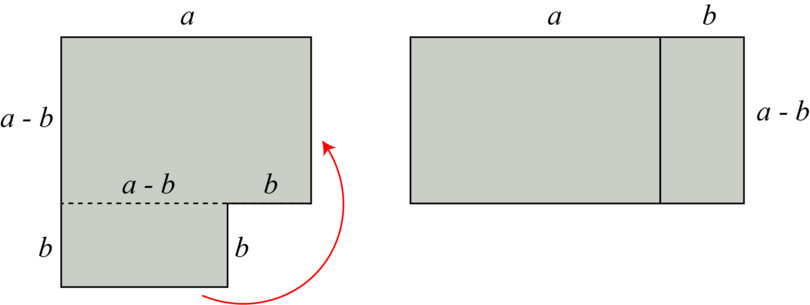The total area of the rectangle on the right can be written as:

Therefore:

Note that the first terms of the two brackets are identical. The last terms differ only in that they have opposite signs.

### Worked example 6.7: Expanding algebraic expressions with two similar brackets that have opposite signs (Method 1)

Expand and simplify:

1. Step 1: Apply the distributive law to the two brackets before distributing the coefficient.

Remember the acronym FOIL:
First term in each bracket
Outside terms
Inside terms
Last term in each bracket

Multiply the two terms that stand first in each bracket.

2. Step 2: Multiply the two terms that stand on the outsides, that is, the furthest away from each other.

3. Step 3: Multiply the two terms that stand on the insides, that is, the closest together.

4. Step 4: Multiply the two terms that stand last in each bracket.

5. Step 5: Combine the answers from Steps 1 to 4.

Notice that when you add the two middle terms, $+2xy$ and $-2xy$, the result is 0.

6. Step 6: Collect like terms.

7. Step 7: Apply the distributive law to the remaining bracket.

\begin{align} \phantom{=} & \, 2(x-2y)(x+2y) \\ =& \, 2(x^2-4y^2)\\ =& \, 2x^2-8y^2 \end{align}

If you understand the method above, you may also use the shorter method shown in the worked example below. Note that the following method can only be applied to two brackets where the first terms are identical and the last terms only differ in that they have opposite signs.

### Worked example 6.8: Expanding algebraic expressions with two similar brackets that have opposite signs (Method 2)

Expand and simplify:

1. Step 1: Apply the distributive law to the two brackets before distributing the coefficient. Square the term that stands first in each bracket.

2. Step 2: Square the term that stands last in each bracket, without taking its sign into account.

3. Step 3: Write down the answers from Steps 1 and 2. Always put a minus sign between them.

4. Step 4: Apply the distributive law to the remaining bracket.

Remember that the minus sign in front of the 4 is part of the coefficient that must be multiplied by each term in the bracket.

\begin{align} &-4(3a+2b)(3a-2b) \\ =&-4(9a^2-4b^2)\\ =&-36a^2+16b^2 \end{align}

When you have two brackets where the first terms are identical and the last terms only differ in that they have opposite signs, then the result of adding the two middle terms will always be 0. This is why you only have to square the first and last terms. The sign between these two terms in the answer must always be a minus sign.

### Exercise 6.5: Expand algebraic expressions with two similar brackets that have opposite signs

Expand and simplify the following algebraic expressions.

1. \begin{align} &-(m-n)(m+n)\\ =&-(m^2-n^2) \\ =&-m^2+n^2 \end{align}
2. \begin{align} \phantom{=} & \, 5(2b-c)(2b+c) \\ =& \, 5(4b^2-c^2) \\ =& \, 20b^2-5c^2 \\ \end{align}
3. \begin{align} \phantom{=} & \, (p+q)(p-q)-2(2p+q)(2p-q) \\ =& \, p^2-q^2 -2(4p^2-q^2) \\ =& \, p^2-q^2-8p^2+2q^2 \\ =& \, -7p^2+q^2 \\ \end{align}
4. \begin{align} \phantom{=} & \, 8d^2-2(3c+4d)(3c-4d) \\ =& \, 8d^2-2(9c^2-16d^2) \\ =& \, 8d^2-18c^2+32d^2 \\ =& \, 40d^2-18c^2 \end{align}

## 6.2 Factorising algebraic expressions

The algebraic expression $a(b+c)$ has two factors: $a$ and $(b+c)$. When we expand the expression, we multiply the two factors using the distributive law: $a(b+c)=ab+ac$. We can reverse this process.

Suppose we start with the expression $ab+ac$. To write the expression as a product of factors, we have to factorise it. This means we have to identify the factors that can be multiplied to give the expression.

The variable $a$ is a common factor of the two terms. We therefore know that it is one of the factors of the expression. If we divide each term by $a$, we are left with $b+c$. Therefore $ab+ac=a(b+c)$

factorise We factorise an algebraic expression by writing it as a product of factors that can be multiplied to give the original expression.

Factorising an algebraic expression is the reverse of expanding it. We can represent the two processes as follows: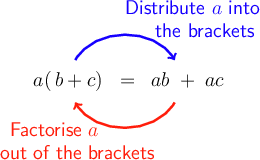### Worked example 6.9: Factorising algebraic expressions (Method 1)

Factorise the expression.

1. Step 1: Find the HCF of the numbers.

Last year you learnt that to find the HCF, you write the two numbers as a product of their prime factors in index form.

$12=2^2\times3$ and $8=2^3$

Then you must identify the prime factors that are contained in both numbers, to the lowest index.

2. Step 2: Find the HCF of the variables.

Apply the same principles you used in Step 1. Identify the variables that are contained in both terms, to the lowest index.

We have $ab$ and $a^2c$.

3. Step 3: Combine the answers from Step 1 and 2 to find the HCF for the full expression.

4. Step 4: Represent each term as the area of a rectangle. The rectangles must have the answer to Step 4 as a common side.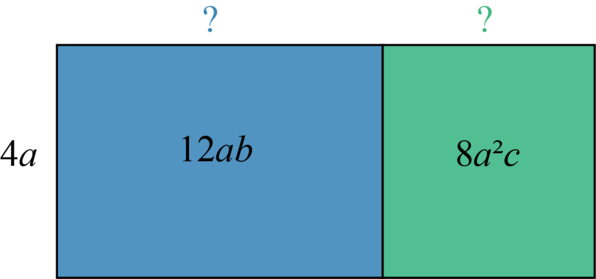5. Step 5: Find the unknown side of each rectangle.

For the blue rectangle, we must multiply the side $4a$ by $3b$ to get an area of $12ab$.

For the green rectangle, we must multiply the side $4a$ by $2ac$ to get an area of $8a^2c$.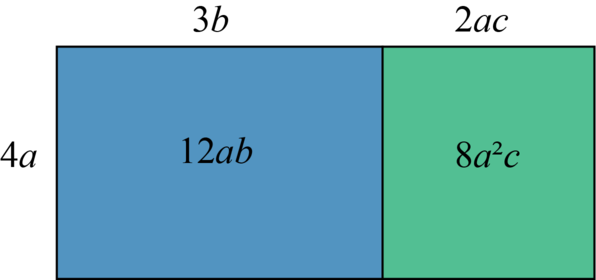6. Step 6: The sides of the combined rectangle give the factors of the original expression.

### Worked example 6.10: Factorising algebraic expressions (Method 2)

Factorise the expression.

1. Step 1: Find the HCF of the numbers.

Write the two numbers in the expression as a product of their prime factors in index form.

$20=2^2\times5$ and $15=3\times5$

Then identify the prime factors that are contained in both numbers, to the lowest index.

2. Step 2: Find the HCF of the variables.

Apply the same principles you used in Step 1. Identify the variables that are contained in both terms, to the lowest index.

We have $x^2y$ and $x^3$.

3. Step 3: Combine the answers from Step 1 and 2 to find the HCF for the full expression. This is the first factor.

4. Step 4: To find the second factor, divide each term by the first factor.

\begin{align} \phantom{=} & \, \frac{20x^2y}{5x^2}-\frac{15x^3}{5x^2} \\ =& \, \frac{20 \times x \times x \times y}{5\times x \times x}-\frac{15\times x \times x \times x}{5\times x} \\ =& \, \frac{\cancel{20}^4 \times \cancel x \times \cancel x \times y}{\cancel{5}^1 \times \cancel x \times \cancel x}-\frac{\cancel{15}^3 \times \cancel x \times \cancel x \times x}{\cancel{5}^1 \times \cancel x \times \cancel x} \\ =& \, 4y-3x \end{align}
5. Step 5: Write down the two factors from Steps 3 and 4.

### Exercise 6.6: Factorise algebraic expressions

1. For each of the following, find the missing factor.

The HCF of $4x$ and $-4$ is $4$.

The missing factor is $4$.

Divide each term by $3a$:

The missing factor is $(b+2c)$.

The HCF of $12xy$ and $16xz$ is $4x$.

The missing factor is $4x$.

Divide each term by $3pq$:

The missing factor is $(3p+5qr)$.

Divide each term by $(a+1)$:

The missing factor is $(x-y)$.

2. Factorise each of the following algebraic expressions.

HCF $=4$

To get the other factor, divide each term inside the bracket by the HCF.

\begin{align} & \, 4x-20y+16z \\ =& \, 4(x-5y+4z) \end{align}
\begin{align} & \, b^2-3ab^2 \\ =& \, b^2(1-3a) \end{align}
\begin{align} &\, 18mn^2+24m^3n \\ =&\, 6mn(3n+4m^2) \end{align}
\begin{align} &\, -16x^2yz-40x^2y+32x^3y^2 \\ =&\, 8x^2y(-2z-5+4xy) \end{align}

You may also choose to take the HCF as $-8x^2y$. If you do, be careful with the signs of the terms in the brackets:
$-16x^2yz-40x^2y+32x^3y^2=-8x^2y(2z+5-4xy)$

\begin{align} &\, 2p(q-4)+3q(q-4) \\ =&\, (q-4)(2p+3q) \end{align}

## 6.3 Simplifying algebraic expressions with fractions

Last year you learnt how to add, subtract, multiply and divide fractions.

To add or subtract fractions with different denominators, you did the following:

1. Find the Lowest Common Denominator (LCD).
2. Find equivalent fractions with the LCD as their denominator.
3. Add or subtract the numerators.
4. Simplify if needed.

Here is an example you did last year:

\begin{align} \phantom{=} & \, \frac{2}{8}+\frac{4}{12}\\ =& \, \frac{6+8}{24} \\ =& \, \frac{14}{24} \\ =& \, \frac{7}{12} \end{align}

To multiply a fractions by another fraction, you did the following:

1. Multiply the numerators.
2. Multiply the denominators.
3. Simplify if needed.

Here is an example you did last year:

\begin{align} \phantom{=} & \, \frac{5}{6}\times \frac{3}{8}\\ =& \, \frac{15}{48} \\ =& \, \frac{5}{16} \\ \end{align}

To divide a fraction by a fraction, you did the following:

1. Find the reciprocal of the fraction you are dividing by.
2. Multiply the two fractions.
3. Simplify if needed.

Here is an example you did last year:

\begin{align} \phantom{=} & \, \frac{3}{4}\div \frac{1}{8}\\ =& \, \frac{3}{4}\times \frac{8}{1}\\ =& \, \frac{24}{4} \\ =& \, 6 \\ \end{align}

We use the same principles when simplifying algebraic expressions that have fractions.

### Worked example 6.11: Simplifying algebraic fractions with whole number denominators

Simplify:

1. Step 1: Find the LCD of the denominators.

To find the LCD, write the two numbers as a product of their prime factors in index form.

$9=3^2\times5$ and $15=3\times5$

Then write down the prime factors, each one to its highest index.

2. Step 2: Find equivalent fractions with the LCD from Step 1 as their denominators.

To make the denominators the same, multiply each denominator by the the factor that will result in the LCD. So you need to multiply the 9 denominator by 5 to get 45, and you need to multiply the 15 denominator by 3 to get 45.

To make sure that you are creating a fraction that is equivalent to the original fraction, you must multiply or divide both the numerator and the denominator by the same number.

\begin{align} \phantom{=} & \, \frac{2x-3y}{9} + \frac{3x-4y}{15} \\ =& \, \frac{2x-3y \; {\color{red}{(\times 5)}} }{9 \; {\color{red}{(\times 5)}}} + \frac{3x-4y \; {\color{red}{(\times 3)}}}{15 \; {\color{red}{(\times 3)}}} \\ =& \, \frac{5(2x-3y) + 3(3x-4y)}{45} \end{align}

When fractions have the same denominator, you can simply add or subtract the numerators.

3. Step 3: If needed, expand the numerators.

\begin{align} \phantom{=} & \, \frac{2x-3y}{9}+\frac{3x-4y}{15} \\ =& \, \frac{5(2x-3y)+3(3x-4y)}{45} \\ =& \, \frac{10x-15y+9x-12y}{45} \end{align}
4. Step 4: Collect like terms and simplify.

\begin{align} \phantom{=} & \, \frac{2x-3y}{9}+\frac{3x-4y}{15} \\ =& \, \frac{5(2x-3y)+3(3x-4y)}{45} \\ =& \, \frac{10x-15y+9x-12y}{45} \\ =& \, \frac{19x-27y}{45} \end{align}

### Worked example 6.12: Simplifying algebraic fractions with variables in their denominators

Simplify:

1. Step 1: Find the LCD of the numbers in the denominators.

The only number in the denominators is 2.

2. Step 2: Find the LCD of the variables in the denominators.

Apply the same principles you use for numbers. Write down the different variables, each to the highest index.

We have $x^2$ and $x$.

3. Step 3: Combine the answers from Steps 1 and 2 to find the full LCD of the denominators.

4. Step 4: Find equivalent fractions with the LCD from Step 3 as their denominator.

Remember that you need to multiply or divide both the numerator and the denominator by the same numbers or variables. Also, when fractions have the same denominator, you can add or subtract the numerators.

\begin{align} \phantom{=} & \, \frac{1 + x}{x^2} + \frac{4}{2x} \\ = & \, \frac{1 + x \; {\color{red}{(\times2)}}}{x^2 \; {\color{red}{(\times 2)}}} + \frac{4 \; {\color{red}{(\times x)}}}{2x \; {\color{red}{(\times x)}}} \\ = & \, \frac{2(1+x)+4(x)}{2x^2} \\ \end{align}
5. Step 5: If needed, expand the numerators.

\begin{align} \phantom{=} & \, \frac{1+x}{x^2}+\frac{4}{2x} \\ =& \, \frac{2(1+x)+4(x)}{2x^2} \\ =& \, \frac{2+2x+4x}{2x^2} \end{align}
6. Step 6: Collect like terms and simplify.

\begin{align} \phantom{=} & \, \frac{1+x}{x^2}+\frac{4}{2x} \\ =& \, \frac{2(1+x)+4(x)}{2x^2} \\ =& \, \frac{2+2x+4x}{2x^2} \\ =& \, \frac{2+6x}{2x^2} \end{align}

### Exercise 6.7: Simplify algebraic expressions with fractions

Simplify the following algebraic expressions.

1. \begin{align} \phantom{=} & \, \frac{2a}{3}+\frac{3b}{4} \\ =& \, \frac{4(2a)+3(3b)}{12} \\ =& \, \frac{8a+9b}{12} \\ \end{align}
2. \begin{align} \phantom{=} & \, \frac{1}{3x}-\frac{2}{3y} \\ =& \, \frac{y(1)-x(2)}{3xy} \\ =& \, \frac{y-2x}{3xy} \\ \end{align}
3. \begin{align} \phantom{=} & \, \frac{2b+3a}{6}-\frac{4a}{5} \\ =& \, \frac{5(2b+3a)-6(4a)}{30} \\ =& \, \frac{10b+15a-24a}{30} \\ =& \, \frac{10b-9a}{30} \end{align}
4. \begin{align} \phantom{=} & \, \frac{6+2p}{8}-\frac{3+5p}{16} \\ =& \, \frac{2(6+2p)-1(3+5p)}{16} \\ =& \, \frac{12+4p-3-5p}{16} \\ =& \, \frac{-p+9}{16} \end{align}
5. \begin{align} \phantom{=} & \, \frac{m+2}{ab}+\frac{3}{a^2} \\ =& \, \frac{a(m+2)+b(3)}{a^2 b} \\ =& \, \frac{am+2a+3b}{a^2b} \\ \end{align}

## 6.4 Practical applications

Algebraic expressions can be very useful when you need to solve problems. You can use variables to represent numbers where the value may vary. Here are some tips that you may find useful:

1. Three consecutive numbers can be represented as: $x,\space x+1, \space x+2$
2. More than, for example 5 more than $x$: $x+5$
3. Less than, for example 5 less than $x$: $x-5$
4. Double or twice, for example double $y$ or twice $y$: $2y$
5. Half, for example half of $y$: $y \div 2$ or $\frac{1}{2}y$
6. Seconds in $m$ minutes and $h$ hours: $60m$ and $3,600h$
7. Kobo in $n$ naira: $100n$
8. Total cost, for example total cost of $p$ pens and $r$ rulers: $p+r$
9. The area of a square is given by $A=s^2$. The perimeter of a square is given by $P=4s$.
10. The area of a rectangle is given by $A=l \times b$. The perimeter of a rectangle is given by $P=2l+2b$.

After you have put together an algebraic expression, expand it if possible and give the answer in its simplest form.

In your answer, state what the variable stands for, for example, "where $$₦\,x$$ is the price of a small sachet of milk", which is used in the exercise below.

### Exercise 6.8: Use algebraic expressions for practical problems

1. A small sachet of milk costs $$₦\,x$$ and a small sachet of noodles costs $$₦\,20$$ more. Work out the cost of 4 sachets of milk and 8 sachets of noodles.

A small sachet of noodles costs $&#8358;\,x+20$.

\begin{align} &\phantom{000}4\times x+8\times (x+20) \\ &=4x+8(x+20) \\ &=4x+8x+160 \\ &=12x+160 \end{align}

The cost is $&#8358;\,12x+160$, where $&#8358;\,x$ is the price of a small sachet of milk.

2. A shop owner sells rice at $$₦\,x$$ per cup. She also sells maize per cup, at half the price of the rice. If she sold 50 cups of rice and 30 cups of maize, what was her income?

The price of her maize is $&#8358;\,\frac{1}{2}x$ per cup.

\begin{align} &\phantom{000}50\times x+30\times \frac{1}{2}x \\ &=50x+15x \\ &=65x \end{align}

The shop owner's income is $&#8358;\,65x$, where $&#8358;\,x$ is the price of one cup of rice.

3. In a family of a father, mother and one daughter, the mother is currently $x$ years old. This is 25 years older than her daughter. In 10 years' time, the daughter's age will be half of her father's age. How old will her father be in 10 years' time?

• The daughter's age is currently $x-25$ years.
• In 10 years' time, the daughter will be $(x-25)+10$ years. We can simplify this to $x-15$ years.
• In 10 years' time, the father's age will be double the daughter's age. This is given by $2(x-15)$. We can simplify it to $2x-30$ years.

The age of the father will be $2x-30$ years, where $x$ is the current age of the mother.

4. The side length of square P is $x$ units. The side length of square Q is 4 units longer than that of square P. Calculate the area of each square.

Square P:
$A=x^2$ square units

Square Q:
$A=(x+4)^2=(x+4)(x+4)=x^2+8x+16$ square units, where $x$ is the side length of square P.

5. The length of a rectangle is 3 cm more than its breadth. Calculate the perimeter and the area of the rectangle.

\begin{align} P&=2l+2b \\ &=2(b+3)+2b \\ &=2b+6+2b \\ &=4b+6 \end{align}

The perimeter of the rectangle is $4b+6\text{ cm}$, where $b \text{ cm}$ is the breadth.

\begin{align} A&=l\times b \\ &=(b+3)\times b \\ &=b(b+3) \\ &=b^2+3b \end{align}

The area of the rectangle is $b^2+3b\text{ cm}^2$, where $b \text{ cm}$ is the breadth.

6. The sum of three consecutive numbers is $y$. If the second of the three numbers is $a$, determine $y$.

The numbers are $a-1$, $a$ and $a+1$.

\begin{align} y&=(a-1)+a+(a+1) \\ &=a-1+a+a+1 \\ &=3a \\ \end{align}

$y=3a$, where $a$ is the second number.

7. In a Mathematics test, Safiyah scored $p$ marks. Nkechi scored 7 marks less than Safiyah. Fadekemi scored twice as many marks as Nkechi. Work out the total mark of the three students.

Safiyah: $p$
Nkechi: $p-7$
Fadekemi: $2\times(p-7)$

\begin{align} &\phantom{000}p+(p-7)+2(p-7) \\ &=p+p-7+2p-14 \\ &=4p-21 \end{align}

Their total mark is $4p-21$, where $p$ is Safiyah's mark.

8. Abodunrin has $$₦\,n$$ and Ikenna has $$₦\,20$$ more. Abodunrin spends 500 kobo and Ikenna spends 750 kobo. Calculate how many kobos they have left altogether.

Abodunrin: $100n-500$
Ikenna: $100(n+20)-750$

\begin{align} &\phantom{000}100n-500+100(n+20)-750 \\ &=100n-500+100n+2,000-750 \\ &=100n+100n-500+2,000-750 \\ &=200n+750 \end{align}

They have $200n+750$ kobo left, where $&#8358;\,n$ is the original amount of money Abodunrin had.

9. Consider the diagram below. Calculate the total area of the two rectangles.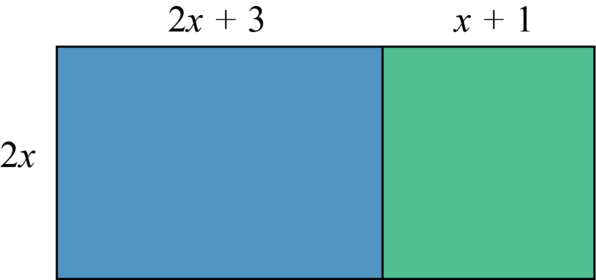\begin{align} A&=l\times b \\ &=(2x+3+x+1)\times2x \\ &=2x(3x+4) \\ &=6x^2+8x \end{align}
10. Rectangle X has a breadth of $2x\text{ cm}$ and a length of $y\text{ cm}$. Rectangle Y has a breadth half as long as the breadth of Rectangle X. The length of Rectangle Y is $10\text{ cm}$ longer than that of Rectangle X. Calculate by how much the area of Rectangle Y is larger than the area of Rectangle X.

Breadth of Rectangle X: $2x$
Length of Rectangle X: $y$
Breadth of Rectangle Y: $2x \div 2=x$
Length of Rectangle Y: $y+10$

\begin{align} A_Y-A_X&=x \times (y+10)-2x\times y \\ &= x(y+10)-2xy\\ &=xy+10x-2xy \\ &=10x-2xy+xy \\ &=10x-xy \end{align}

The area of Rectangle Y is $10x-xy\text{ cm}$ larger than the area of Rectangle X.

## 6.5 Summary

• An algebraic expression is a mathematical statement that contains a combination of numbers, symbols, variables and mathematical operators. It does not have an equals sign.
• A term can be a single number (positive or negative), or a variable, or one or more numbers and variables that are being multiplied together. Terms are separated by $+$ or $âˆ’$ signs.
• A coefficient is a number multiplied by a variable in a single term of an algebraic expression.
• A constant is a term in an algebraic expression that does not contain a variable.
• We expand an algebraic expression by using the distributive law to remove brackets and then collecting like terms.
• The distributive law states that the multiplying factor or coefficient of a bracket can be distributed across each of the terms inside the bracket, for example:
• $a(b+c)=ab+bc$
• $(a+b)(c+d)=ac+ad+bc+bd$
• $(a+b)^2=a^2+2ab+b^2$
• $(a+b)(a-b)=a^2-b^2$
• We factorise an algebraic expression by writing it as a product of factors that can be multiplied to give the original expression. Factorising an algebraic expression is the reverse of expanding an algebraic expression.
• We can factorise an algebraic expression by identifying the HCF of all the terms, for example:
• $15x+25y=5(3x+5y)$
• $a^2b+ab^2=ab(a+b)$
• For algebraic expressions that contain fractions, we find the LCD of the denominators and then add or subtract.
• We can use algebraic expressions to solve problems. The answer must be given in its simplest form.